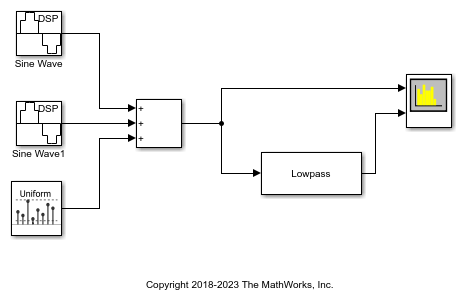# Obtain Measurements Data Programmatically for Spectrum Analyzer Block

Compute and display the power spectrum of a noisy sinusoidal input signal using the Spectrum Analyzer block. Measure the peaks, cursor placements, adjacent channel power ratio, distortion, and CCDF values in the spectrum by enabling these block configuration properties:

• PeakFinder

• CursorMeasurements

• ChannelMeasurements

• DistortionMeasurements

• CCDFMeasurements

### Open and Inspect the Model

Filter a streaming noisy sinusoidal input signal using a Lowpass Filter block. The input signal consists of two sinusoidal tones: 1 kHz and 15 kHz. The noise is white Gaussian noise with zero mean and a variance of 0.05. The sampling frequency is 44.1 kHz. Open the model and inspect the various block settings.

```model = 'spectrumanalyzer_measurements.slx'; open_system(model) ```Access the configuration properties of the Spectrum Analyzer block using the `get_param` function.

```sablock = 'spectrumanalyzer_measurements/Spectrum Analyzer'; cfg = get_param(sablock,'ScopeConfiguration'); ```

### Enable Measurements Data

To obtain the measurements, set the `Enable` property of the measurements to `true`.

```cfg.CursorMeasurements.Enable = true; cfg.ChannelMeasurements.Enable = true; cfg.PeakFinder.Enable = true; cfg.DistortionMeasurements.Enable = true; ```

### Simulate the Model

Run the model. The Spectrum Analyzer block compares the original spectrum with the filtered spectrum.

```sim(model) ```The right side of the spectrum analyzer shows the enabled measurement panes.

### Using `getMeasurementsData`

Use the `getMeasurementsData` function to obtain these measurements programmatically.

```data = getMeasurementsData(cfg) ```
```data = 1x5 table SimulationTime PeakFinder CursorMeasurements ChannelMeasurements DistortionMeasurements ______________ __________ __________________ ___________________ ______________________ {[0.9985]} 1x1 struct 1x1 struct 1x1 struct 1x1 struct ```

The values shown in measurement panes match the values shown in `data`. You can access the individual fields of `data` to obtain the various measurements programmatically.

### Compare Peak Values

As an example, compare the peak values. Verify that the peak values obtained by `data.PeakFinder` match with the values seen in the `Spectrum Analyzer` window.

```peakvalues = data.PeakFinder.Value frequencieskHz = data.PeakFinder.Frequency/1000 ```
```peakvalues = 26.9076 26.2675 -2.9136 frequencieskHz = 15.0015 1.0049 16.3796 ```

### Save and Close the Model

```save_system(model); close_system(model); ```